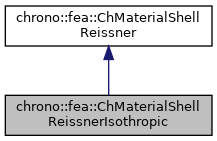chrono::fea::ChMaterialShellReissnerIsothropic Class Reference

## Description

Material definition.

This class implements material properties for a layer from the Reissner theory, for the case of isotropic linear linear elastic material. This is probably the material that you need most often when using 6-field shells.

#include <ChMaterialShellReissner.h>

Inheritance diagram for chrono::fea::ChMaterialShellReissnerIsothropic:[legend]
Collaboration diagram for chrono::fea::ChMaterialShellReissnerIsothropic:[legend]

## Public Member Functions

ChMaterialShellReissnerIsothropic (double rho, double E, double nu, double alpha=1.0, double beta=0.1)
Construct an isotropic material. More...

double Get_E () const
Return the elasticity moduli.

double Get_nu () const
Return the Poisson ratio.

double Get_alpha () const
Return the shear factor.

double Get_beta () const
Return the torque factor.

virtual void ComputeStress (ChVector<> &n_u, ChVector<> &n_v, ChVector<> &m_u, ChVector<> &m_v, const ChVector<> &eps_u, const ChVector<> &eps_v, const ChVector<> &kur_u, const ChVector<> &kur_v, const double z_inf, const double z_sup, const double angle)
The FE code will evaluate this function to compute per-unit-length forces/torques given the u,v strains/curvatures. More...

virtual void ComputeTangentC (ChMatrixRef mC, const ChVector<> &eps_u, const ChVector<> &eps_v, const ChVector<> &kur_u, const ChVector<> &kur_v, const double z_inf, const double z_sup, const double angle)
Compute [C] , that is [ds/de], the tangent of the constitutive relation per-unit-length forces/torques vs strains. More...Public Member Functions inherited from chrono::fea::ChMaterialShellReissner
ChMaterialShellReissner ()
Construct an isotropic material.

double Get_rho () const
Return the material density.Protected Attributes inherited from chrono::fea::ChMaterialShellReissner
double m_rho
density

## Constructor & Destructor Documentation

 chrono::fea::ChMaterialShellReissnerIsothropic::ChMaterialShellReissnerIsothropic ( double rho, double E, double nu, double alpha = 1.0, double beta = 0.1 )

Construct an isotropic material.

Parameters
 rho material density E Young's modulus nu Poisson ratio alpha shear factor beta torque factor

## Member Function Documentation

 void chrono::fea::ChMaterialShellReissnerIsothropic::ComputeStress ( ChVector<> & n_u, ChVector<> & n_v, ChVector<> & m_u, ChVector<> & m_v, const ChVector<> & eps_u, const ChVector<> & eps_v, const ChVector<> & kur_u, const ChVector<> & kur_v, const double z_inf, const double z_sup, const double angle )
virtual

The FE code will evaluate this function to compute per-unit-length forces/torques given the u,v strains/curvatures.

Parameters
 n_u forces along u direction (per unit length) n_v forces along v direction (per unit length) m_u torques along u direction (per unit length) m_v torques along v direction (per unit length) eps_u strains along u direction eps_v strains along v direction kur_u curvature along u direction kur_v curvature along v direction z_inf layer lower z value (along thickness coord) z_sup layer upper z value (along thickness coord) angle layer angle respect to x (if needed) -not used in this, isotropic

Implements chrono::fea::ChMaterialShellReissner.

 void chrono::fea::ChMaterialShellReissnerIsothropic::ComputeTangentC ( ChMatrixRef mC, const ChVector<> & eps_u, const ChVector<> & eps_v, const ChVector<> & kur_u, const ChVector<> & kur_v, const double z_inf, const double z_sup, const double angle )
virtual

Compute [C] , that is [ds/de], the tangent of the constitutive relation per-unit-length forces/torques vs strains.

Parameters
 mC tangent matrix eps_u strains along u direction eps_v strains along v direction kur_u curvature along u direction kur_v curvature along v direction z_inf layer lower z value (along thickness coord) z_sup layer upper z value (along thickness coord) angle layer angle respect to x (if needed) -not used in this, isotropic

Reimplemented from chrono::fea::ChMaterialShellReissner.